# Fourier-Stieltjes algebra

Letbe an arbitrary locally compact group. For, let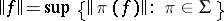, whereis the set of all equivalence classes of unitary continuous representations of(cf. also Unitary representation). The completion of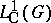with respect to this norm is a Banach algebra, denoted byand called the full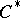-algebra of. Ifis Abelian andits dual group, thenis isometrically isomorphic to the Banach algebra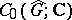of all complex-valued continuous functions on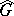vanishing at infinity.

Let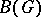be the complex linear span of the set of all continuous positive-definite functions on.

1) The-vector spaceis isomorphic to the dual space of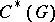. With the dual norm and the pointwise product on,is a commutative Banach algebra [a4].

This Banach algebra is called the Fourier–Stieltjes algebra of. Ifis Abelian, thenis isometrically isomorphic to the Banach algebra of all bounded Radon measures on.

2) On the boundary of the unit ball of(i.e. on) the weak topology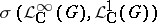coincides with the compact-open topology on([a3]; see also [a9], [a6]).

3) The following properties are satisfied ([a4]):

a) The Fourier algebra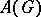is a closed ideal of;

b)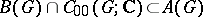;

c)coincides with the closure inof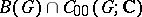;

d), with equality of the corresponding norms. Here,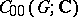is the algebra of functions of compact support on. In [a14], M.E. Walter showed that(and also) completely characterizes. More precisely, assume that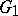andare locally compact groups; then the following assertions are equivalent:

the locally compact groupsandare topologically isomorphic;

the Banach algebrasand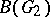are isometrically isomorphic;

the Banach algebras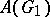and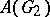are isometrically isomorphic.

He also gave a description of the dual of.

For a connected semi-simple Lie group, M. Cowling [a1] has given a description of the spectrum of; surprisingly, ifis Abelian, then the spectrum ofseems to be much more complicated than in the non-Abelian case!

Ifis amenable, then ([a3])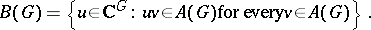(a1)

V. Losert [a12] proved the converse assertion: if (a1) holds, thenmust be amenable!

In a difficult paper [a7], C.S. Herz tried to extend the preceding results, replacing unitary representations by representations in Banach spaces. He partially succeeded in the amenable case. See also [a2], [a5].

M. Lefranc generalized Paul Cohen's idempotent theorem tofor arbitrary locally compact groups([a10], [a8]; see also [a11] for detailed proofs).